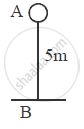Advertisement Remove all ads

# A Body of Mass 10 Kg is Kept at a Height of 5m. It is Allowed to Fall and Reach the Ground. (I) What is the Total Mechanical Energy Possessed by the Body at the Height of 2m Assuming - Physics

Short Note

A body of mass 10 Kg is kept at a height of 5m. It is allowed to fall and reach the ground.
(i) What is the total mechanical energy possessed by the body at the height of 2m assuming it is a frictionless medium ?
(ii) What is the kinetic energy possessed by the body just before hitting the ground ? Take g = 10 m/s2.

Advertisement Remove all ads

#### Solution

m = 10 kg
h = 5 m
g = 10 m/s²
Mechanical Energy = Potential Energy + Kinetic Energy
Potential Energy at h of 5 m  = mgh = (10) ×(10) × (5) = 500 J

i) Energy neither can be created or destroyed but can be converter in to another form. as its a Frictionless surface so no energy loss in any other form

so

Total Mechanical Energy at h of 2m = 500  J

ii) Just Before hitting the ground  h = 0 m

so potential energy = 0 J

Total Mechanical Energy = 500 J

500 = 0 + Kinetic Energy

=> Kinetic Energy = 500 J

or we can find it by using

KE = (1/2)mV²

V² - U² = 2aS   U = 0  S = 5 ,  a = g = 10m/(s²)

V² = 2 × 10 × 5= 100

KE = (1/2)× 10 × 100 = 500 J

in part i) if we need to found  Kinetic energy & Potential energy separately

Potential energy at 2 m = 10 × 10 × 2 = 200 J

Kinetic Energy = Total energy - potential energy = 500 - 200 = 300 J

or we can calculate by

Kinetic Energy = (1/2) m V²

2aS = V² - U²

U = 0  , S = 5 -2 = 3 m  a = g = 10m/(s²)

=> 2×10×3 = V²

=> V² = 60

Kinetic Energy = (1/2) 10 × 60 = 300 JConcept: Principle of Conservation of Energy
Is there an error in this question or solution?
Advertisement Remove all ads

#### APPEARS IN

Advertisement Remove all ads
Advertisement Remove all ads
Share
Notifications

View all notifications

Forgot password?
Course